# Differential calculus facts for kids

Kids Encyclopedia Facts

Differential calculus, a branch of calculus, is the study of finding out the rate of change of a variable compared to another variable, by using functions. It is a way to find out how a shape changes from one point to the next, without needing to divide the shape into an infinite number of pieces. Differential calculus is the opposite of integral calculus. It was developed in the 1670s and 1680s by Sir Isaac Newton and Gottfried Leibniz.

## Background

Unlike a number such as 5 or 200, a variable can change its value. For example, distance and time are variables. At an Olympic running race, as the person runs, their distance from the starting line goes up. Meanwhile, a stopwatch or clock measures the time as it goes up. We can measure the average speed of the runner, if we divide the distance they travelled by the time it took. But this does not say what speed the person was running at exactly 1.5 seconds into the race. If we had the distance at 1 second and the distance at 2 seconds, we would still only have an average, although it would probably be more correct than the average for the whole race.

Until calculus was invented, the only way to work this out was to cut the time into smaller and smaller pieces, so the average speed over the smaller time would get closer and closer to the actual speed at exactly 1.5 seconds. This was a very long and hard process, and had to be done each time people wanted to work something out. Certainly it is much more difficult for a driver to figure out a car's speed by using only its odometer (distance meter) and clock—without a speedometer.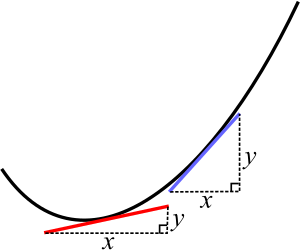On a curve, two different points have different slopes. The red and blue lines are tangents to the curve.

A very similar problem is to find the slope (how steep it is) at any point on a curve. The slope of a straight line is easy to work out—it is simply how much it goes up (y or vertical) divided by how much it goes across (x or horizontal). If a line is parallel to the x axis, then its slope is zero. If a straight line went through (x,y) = (2,10) and (4,18), the line goes up 8 and goes across 2, so its slope is 8 divide 2, which is 4.

On a 'curve', though, the slope is a variable (has different values at different points), because the line bends. But if the curve was to be cut into very, very small pieces, the curve at the point would look almost like a very short straight line. So to work out its slope, a straight line can be drawn through the point with the same slope as the curve at that point. If it is done exactly right, the straight line will have the same slope as the curve, and is called a tangent. But there is no way to know (without calculus) whether the tangent is exactly right, and our eyes are not accurate enough to be certain whether it is exact or simply very close.

What Newton and Leibniz found was a way to work out the slope (or the speed in the distance example) exactly, by using simple and logical rules. They divided the curve into an infinite number of very small pieces. They then chose points on either side of the point they were interested in and worked out tangents at each. As the points moved closer together towards the point they were interested in, the slope approached a particular value as the tangents approached the real slope of the curve. They said that this particular value it approached was the actual slope.

## How it works

Let's say we have a function y = f(x). f is short for function, so this equation means "y is a function of x". This tells us that how high y is on the vertical axis depends on what x (the horizontal axis) is at that time. For example, with the equation y = x², we know that if x is 1, then y will be 1; if x is 3, then y will be 9; if x is 20, then y will be 400.

Choose a point A on the curve, and call its horizontal position x. Then choose another point B on the curve which is a little bit farther across than A, and call its horizontal position x + h. It does not matter how much h is; it is a very small number.

So when we go from point A to point B, the vertical position has gone from f(x) to f(x + h), and the horizontal position has gone from x to x + h. Now, remember that the slope is how much it goes up divided by how much it goes across. So the slope will be: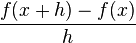$\frac{f(x + h) - f(x)}{h}$

If you bring B closer and closer to A – which means h gets closer and closer to 0 – then we get closer to knowing what the slope is at the point A.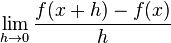$\lim_{h\rightarrow0} \frac{f(x + h) - f(x)}{h}$

Now let's go back to y = x². The slope of this can be determined as follows:\begin{align} &= \lim_{h\rightarrow0} \frac{f(x+h) - f(x)}{h} \\ &= \lim_{h\rightarrow0} \frac{(x+h)^2 - (x)^2}{h} \end{align}

Applying the binomial theorem which states in part that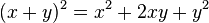$(x+y)^2 = x^2 + 2xy + y^2$, we can reduce the expression to: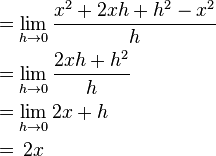\begin{align} &= \lim_{h\rightarrow0} \frac{x^2 + 2xh + h^2 - x^2}{h} \\ &= \lim_{h\rightarrow0} \frac{2xh + h^2}{h} \\ &= \lim_{h\rightarrow0} 2x + h \\ &= \frac{}{} 2x \end{align}

So we know without having to draw any tangent lines that at any point on the curve f(x) = x², the derivative f'(x) (marked with an apostrophe) will be 2x at any point. This process of working out a slope using limits is called differentiation, or finding the derivative.

Leibniz came to the same result, but called h "dx", which means "a tiny amount of x". He called the resulting change in f(x) "dy", which means "a tiny amount of y". Leibniz's notation is used by more books because it is easy to understand, when the equations become more complicated. In Leibniz notation: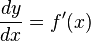$\frac{dy}{dx} = f'(x)$

## Rules

Using the above system, mathematicians have worked out rules which work all the time, no matter which function is being looked at. (Note: here,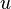$u$ and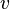$v$ are both functions of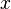$x$)

Condition Function Derivative Example Derivative
A number by itself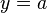$y = a$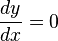$\frac{dy}{dx} = 0$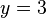$y = 3$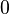$0$
A straight line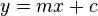$y = mx + c$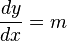$\frac{dy}{dx} = m$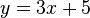$y = 3x + 5$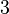$3$
x to the power of a number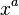$x^a$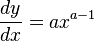$\frac{dy}{dx} = a x^{a-1}$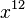$x^{12}$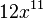$12x^{11}$
A number multiplied by a function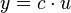$y = c\cdot u$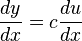$\frac{dy}{dx} = c \frac{du}{dx}$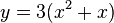$y=3(x^2 + x)$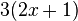$3(2x + 1)$
A function plus another function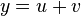$y = u + v$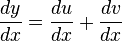$\frac{dy}{dx} = \frac{du}{dx} + \frac{dv}{dx}$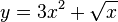$y = 3x^2 + \sqrt{x}$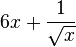$6x + \frac{1}{\sqrt{x}}$
A function minus another function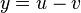$y = u - v$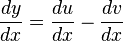$\frac{dy}{dx} = \frac{du}{dx} - \frac{dv}{dx}$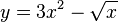$y = 3x^2 - \sqrt{x}$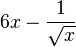$6x - \frac{1}{\sqrt{x}}$
Product Rule
A function multiplied by another function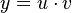$y = u \cdot v$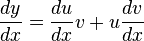$\frac{dy}{dx} = \frac{du}{dx}v + u\frac{dv}{dx}$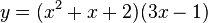$y = (x^2 + x + 2)(3x-1)$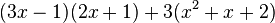$(3x-1)(2x+1) + 3(x^2+x+2)$
Quotient Rule
A function divided by another function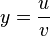$y = \frac{u}{v}$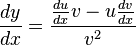$\frac{dy}{dx} = \frac{\frac{du}{dx}v - u\frac{dv}{dx}}{v^2}$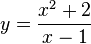$y = \frac{x^2 + 2}{x-1}$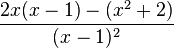$\frac{2x(x - 1) - (x^2 + 2)}{(x-1)^2}$
Chain rule
Used for composite functions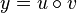$y = u \circ v$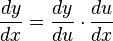$\frac{dy}{dx} = \frac{dy}{du} \cdot \frac{du}{dx}$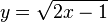$y = \sqrt{2x-1}$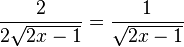$\frac{2}{2\sqrt{2x-1}} = \frac{1}{\sqrt{2x-1}}$
An exponential function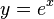$\frac{}{} y = e^x$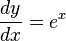$\frac{dy}{dx} = e^x$$\frac{}{} y = e^x$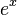$\frac{}{} e^x$

## Images for kidsDifferential calculus Facts for Kids. Kiddle Encyclopedia.